# Mean State

Period Mean (original grids) [K]
Model Period Mean (intersection) [K]
Model Period Mean (complement) [K]
Benchmark Period Mean (intersection) [K]
Benchmark Period Mean (complement) [K]
Bias [K]
RMSE [K]
Phase Shift [months]
Bias Score 
RMSE Score 
Seasonal Cycle Score 
Spatial Distribution Score 
Interannual Variability Score 
Overall Score 
Benchmark [-] 304.
CRUNCEPv7 [-] 303. 303. 303. 304. 304. -0.232 1.36 0.663 0.640 0.511 0.909 0.995 0.757 0.720
GSWP3v1 [-] 305. 305. 304. 304. 304. 1.74 2.05 0.327 0.302 0.646 0.958 0.997 0.803 0.725
Period Mean (original grids) [K]
Model Period Mean (intersection) [K]
Model Period Mean (complement) [K]
Benchmark Period Mean (intersection) [K]
Benchmark Period Mean (complement) [K]
Bias [K]
RMSE [K]
Phase Shift [months]
Bias Score 
RMSE Score 
Seasonal Cycle Score 
Spatial Distribution Score 
Interannual Variability Score 
Overall Score 
Benchmark [-] 307.
CRUNCEPv7 [-] 307. 307. 305. 307. 305. 0.364 1.90 0.228 0.735 0.574 0.983 0.996 0.696 0.760
GSWP3v1 [-] 309. 309. 305. 307. 305. 1.99 2.21 0.175 0.491 0.761 0.986 0.996 0.882 0.813
Period Mean (original grids) [K]
Model Period Mean (intersection) [K]
Model Period Mean (complement) [K]
Benchmark Period Mean (intersection) [K]
Benchmark Period Mean (complement) [K]
Bias [K]
RMSE [K]
Phase Shift [months]
Bias Score 
RMSE Score 
Seasonal Cycle Score 
Spatial Distribution Score 
Interannual Variability Score 
Overall Score 
Benchmark [-] 301.
CRUNCEPv7 [-] 302. 302. 300. 301. 302. 0.706 1.89 0.645 0.602 0.576 0.920 0.997 0.858 0.755
GSWP3v1 [-] 303. 303. 300. 301. 302. 1.80 2.11 0.314 0.436 0.727 0.962 0.998 0.835 0.781
Period Mean (original grids) [K]
Model Period Mean (intersection) [K]
Model Period Mean (complement) [K]
Benchmark Period Mean (intersection) [K]
Benchmark Period Mean (complement) [K]
Bias [K]
RMSE [K]
Phase Shift [months]
Bias Score 
RMSE Score 
Seasonal Cycle Score 
Spatial Distribution Score 
Interannual Variability Score 
Overall Score 
Benchmark [-] 266.
CRUNCEPv7 [-] 263. 263. 266. 266. 269. -2.27 2.97 0.0353 0.867 0.897 0.998 0.989 0.876 0.920
GSWP3v1 [-] 266. 266. 270. 266. 269. 0.485 1.77 0.0300 0.944 0.910 0.998 0.999 0.899 0.943
Period Mean (original grids) [K]
Model Period Mean (intersection) [K]
Model Period Mean (complement) [K]
Benchmark Period Mean (intersection) [K]
Benchmark Period Mean (complement) [K]
Bias [K]
RMSE [K]
Phase Shift [months]
Bias Score 
RMSE Score 
Seasonal Cycle Score 
Spatial Distribution Score 
Interannual Variability Score 
Overall Score 
Benchmark [-] 304.
CRUNCEPv7 [-] 301. 301. 301. 304. 303. -2.52 2.60 1.48 0.0894 0.479 0.770 0.886 0.764 0.578
GSWP3v1 [-] 305. 305. 304. 304. 303. 1.24 1.78 1.04 0.173 0.514 0.845 0.904 0.810 0.627
Period Mean (original grids) [K]
Model Period Mean (intersection) [K]
Model Period Mean (complement) [K]
Benchmark Period Mean (intersection) [K]
Benchmark Period Mean (complement) [K]
Bias [K]
RMSE [K]
Phase Shift [months]
Bias Score 
RMSE Score 
Seasonal Cycle Score 
Spatial Distribution Score 
Interannual Variability Score 
Overall Score 
Benchmark [-] 276.
CRUNCEPv7 [-] 274. 274. 276. 276. 278. -1.79 2.23 0.00705 0.887 0.922 1.00 0.992 0.967 0.948
GSWP3v1 [-] 276. 276. 278. 276. 278. 0.315 1.09 0.00657 0.961 0.944 1.00 1.00 0.972 0.970
Period Mean (original grids) [K]
Model Period Mean (intersection) [K]
Model Period Mean (complement) [K]
Benchmark Period Mean (intersection) [K]
Benchmark Period Mean (complement) [K]
Bias [K]
RMSE [K]
Phase Shift [months]
Bias Score 
RMSE Score 
Seasonal Cycle Score 
Spatial Distribution Score 
Interannual Variability Score 
Overall Score 
Benchmark [-] 286.
CRUNCEPv7 [-] 285. 285. 290. 286. 289. -0.406 1.96 0.0844 0.869 0.888 0.994 0.993 0.937 0.928
GSWP3v1 [-] 287. 286. 291. 286. 289. 0.928 1.61 0.0534 0.870 0.927 0.996 1.00 0.944 0.944
Period Mean (original grids) [K]
Model Period Mean (intersection) [K]
Model Period Mean (complement) [K]
Benchmark Period Mean (intersection) [K]
Benchmark Period Mean (complement) [K]
Bias [K]
RMSE [K]
Phase Shift [months]
Bias Score 
RMSE Score 
Seasonal Cycle Score 
Spatial Distribution Score 
Interannual Variability Score 
Overall Score 
Benchmark [-] 302.
CRUNCEPv7 [-] 302. 302. 302. 302. 304. -0.205 1.75 0.260 0.695 0.739 0.973 0.999 0.828 0.829
GSWP3v1 [-] 304. 304. 304. 302. 304. 1.33 1.91 0.185 0.602 0.814 0.981 0.999 0.915 0.854
Period Mean (original grids) [K]
Model Period Mean (intersection) [K]
Model Period Mean (complement) [K]
Benchmark Period Mean (intersection) [K]
Benchmark Period Mean (complement) [K]
Bias [K]
RMSE [K]
Phase Shift [months]
Bias Score 
RMSE Score 
Seasonal Cycle Score 
Spatial Distribution Score 
Interannual Variability Score 
Overall Score 
Benchmark [-] 268.
CRUNCEPv7 [-] 265. 265. 265. 268. 268. -2.53 3.11 0.0363 0.850 0.893 0.998 0.992 0.910 0.922
GSWP3v1 [-] 267. 267. 269. 268. 268. -0.295 1.48 0.0122 0.962 0.918 0.999 1.00 0.912 0.951
Period Mean (original grids) [K]
Model Period Mean (intersection) [K]
Model Period Mean (complement) [K]
Benchmark Period Mean (intersection) [K]
Benchmark Period Mean (complement) [K]
Bias [K]
RMSE [K]
Phase Shift [months]
Bias Score 
RMSE Score 
Seasonal Cycle Score 
Spatial Distribution Score 
Interannual Variability Score 
Overall Score 
Benchmark [-] 301.
CRUNCEPv7 [-] 302. 302. 296. 301. 299. 0.303 1.79 0.148 0.740 0.825 0.989 0.981 0.878 0.873
GSWP3v1 [-] 303. 303. 297. 301. 299. 1.80 2.04 0.119 0.668 0.878 0.991 0.997 0.906 0.886
Period Mean (original grids) [K]
Model Period Mean (intersection) [K]
Model Period Mean (complement) [K]
Benchmark Period Mean (intersection) [K]
Benchmark Period Mean (complement) [K]
Bias [K]
RMSE [K]
Phase Shift [months]
Bias Score 
RMSE Score 
Seasonal Cycle Score 
Spatial Distribution Score 
Interannual Variability Score 
Overall Score 
Benchmark [-] 286.
CRUNCEPv7 [-] 284. 284. 286. 286. 289. -1.17 1.79 0.267 0.823 0.889 0.982 0.995 0.925 0.917
GSWP3v1 [-] 286. 286. 287. 286. 289. 0.500 1.28 0.156 0.881 0.906 0.989 0.997 0.943 0.937
Period Mean (original grids) [K]
Model Period Mean (intersection) [K]
Model Period Mean (complement) [K]
Benchmark Period Mean (intersection) [K]
Benchmark Period Mean (complement) [K]
Bias [K]
RMSE [K]
Phase Shift [months]
Bias Score 
RMSE Score 
Seasonal Cycle Score 
Spatial Distribution Score 
Interannual Variability Score 
Overall Score 
Benchmark [-] 292.
CRUNCEPv7 [-] 287. 292. 247. 292. 295. -0.605 2.14 0.280 0.732 0.767 0.966 0.992 0.865 0.848
GSWP3v1 [-] 289. 293. 252. 292. 295. 1.14 1.82 0.164 0.688 0.837 0.982 0.997 0.910 0.875
Period Mean (original grids) [K]
Model Period Mean (intersection) [K]
Model Period Mean (complement) [K]
Benchmark Period Mean (intersection) [K]
Benchmark Period Mean (complement) [K]
Bias [K]
RMSE [K]
Phase Shift [months]
Bias Score 
RMSE Score 
Seasonal Cycle Score 
Spatial Distribution Score 
Interannual Variability Score 
Overall Score 
Benchmark [-] 303.
CRUNCEPv7 [-] 304. 304. 301. 303. 303. 1.50 2.34 0.200 0.746 0.824 0.983 0.988 0.826 0.865
GSWP3v1 [-] 304. 304. 301. 303. 303. 1.71 2.09 0.112 0.736 0.893 0.991 0.998 0.912 0.904
Period Mean (original grids) [K]
Model Period Mean (intersection) [K]
Model Period Mean (complement) [K]
Benchmark Period Mean (intersection) [K]
Benchmark Period Mean (complement) [K]
Bias [K]
RMSE [K]
Phase Shift [months]
Bias Score 
RMSE Score 
Seasonal Cycle Score 
Spatial Distribution Score 
Interannual Variability Score 
Overall Score 
Benchmark [-] 268.
CRUNCEPv7 [-] 267. 267. 264. 268. 270. -1.79 2.60 0.0185 0.869 0.875 0.999 1.00 0.889 0.918
GSWP3v1 [-] 269. 269. 268. 268. 270. 0.304 1.58 0.0152 0.942 0.902 0.999 1.00 0.911 0.943
Period Mean (original grids) [K]
Model Period Mean (intersection) [K]
Model Period Mean (complement) [K]
Benchmark Period Mean (intersection) [K]
Benchmark Period Mean (complement) [K]
Bias [K]
RMSE [K]
Phase Shift [months]
Bias Score 
RMSE Score 
Seasonal Cycle Score 
Spatial Distribution Score 
Interannual Variability Score 
Overall Score 
Benchmark [-] 302.
CRUNCEPv7 [-] 300. 299. 301. 302. 303. -2.32 2.81 0.278 0.426 0.699 0.974 1.00 0.865 0.777
GSWP3v1 [-] 303. 303. 303. 302. 303. 1.40 2.07 0.283 0.533 0.743 0.973 0.986 0.906 0.814
Period Mean (original grids) [K]
Model Period Mean (intersection) [K]
Model Period Mean (complement) [K]
Benchmark Period Mean (intersection) [K]
Benchmark Period Mean (complement) [K]
Bias [K]
RMSE [K]
Phase Shift [months]
Bias Score 
RMSE Score 
Seasonal Cycle Score 
Spatial Distribution Score 
Interannual Variability Score 
Overall Score 
Benchmark [-] 292.
CRUNCEPv7 [-] 290. 290. 296. 292. 297. -1.79 2.01 0.0675 0.813 0.908 0.995 1.00 0.930 0.926
GSWP3v1 [-] 293. 293. 299. 292. 297. 0.963 1.27 0.0711 0.882 0.934 0.995 0.997 0.963 0.951
Period Mean (original grids) [K]
Model Period Mean (intersection) [K]
Model Period Mean (complement) [K]
Benchmark Period Mean (intersection) [K]
Benchmark Period Mean (complement) [K]
Bias [K]
RMSE [K]
Phase Shift [months]
Bias Score 
RMSE Score 
Seasonal Cycle Score 
Spatial Distribution Score 
Interannual Variability Score 
Overall Score 
Benchmark [-] 279.
CRUNCEPv7 [-] 278. 278. 279. 279. 282. -1.67 2.11 0.0338 0.859 0.906 0.998 0.997 0.940 0.934
GSWP3v1 [-] 280. 280. 281. 279. 282. 0.566 1.19 0.0362 0.930 0.926 0.998 0.998 0.959 0.956
Period Mean (original grids) [K]
Model Period Mean (intersection) [K]
Model Period Mean (complement) [K]
Benchmark Period Mean (intersection) [K]
Benchmark Period Mean (complement) [K]
Bias [K]
RMSE [K]
Phase Shift [months]
Bias Score 
RMSE Score 
Seasonal Cycle Score 
Spatial Distribution Score 
Interannual Variability Score 
Overall Score 
Benchmark [-] 291.
CRUNCEPv7 [-] 290. 290. 291. 291. 292. -1.07 2.13 0.0518 0.846 0.858 0.996 0.997 0.935 0.915
GSWP3v1 [-] 292. 292. 293. 291. 292. 1.22 1.73 0.0461 0.847 0.907 0.997 0.999 0.940 0.933
Period Mean (original grids) [K]
Model Period Mean (intersection) [K]
Model Period Mean (complement) [K]
Benchmark Period Mean (intersection) [K]
Benchmark Period Mean (complement) [K]
Bias [K]
RMSE [K]
Phase Shift [months]
Bias Score 
RMSE Score 
Seasonal Cycle Score 
Spatial Distribution Score 
Interannual Variability Score 
Overall Score 
Benchmark [-] 304.
CRUNCEPv7 [-] 303. 303. 302. 304. 304. -0.756 1.40 0.719 0.519 0.524 0.901 0.986 0.832 0.714
GSWP3v1 [-] 306. 306. 304. 304. 304. 1.64 1.95 0.380 0.246 0.646 0.949 1.00 0.903 0.732
Period Mean (original grids) [K]
Model Period Mean (intersection) [K]
Model Period Mean (complement) [K]
Benchmark Period Mean (intersection) [K]
Benchmark Period Mean (complement) [K]
Bias [K]
RMSE [K]
Phase Shift [months]
Bias Score 
RMSE Score 
Seasonal Cycle Score 
Spatial Distribution Score 
Interannual Variability Score 
Overall Score 
Benchmark [-] 301.
CRUNCEPv7 [-] 301. 301. 298. 301. 297. -0.000935 1.50 0.766 0.717 0.611 0.887 0.999 0.791 0.769
GSWP3v1 [-] 303. 303. 300. 301. 297. 1.71 1.87 0.285 0.484 0.775 0.964 0.999 0.917 0.819
Period Mean (original grids) [K]
Model Period Mean (intersection) [K]
Model Period Mean (complement) [K]
Benchmark Period Mean (intersection) [K]
Benchmark Period Mean (complement) [K]
Bias [K]
RMSE [K]
Phase Shift [months]
Bias Score 
RMSE Score 
Seasonal Cycle Score 
Spatial Distribution Score 
Interannual Variability Score 
Overall Score 
Benchmark [-] 290.
CRUNCEPv7 [-] 290. 290. 291. 290. 289. -0.559 2.32 0.378 0.583 0.703 0.951 0.996 0.864 0.800
GSWP3v1 [-] 292. 292. 293. 290. 289. 1.67 2.39 0.277 0.556 0.761 0.964 0.995 0.916 0.826

# Temporally integrated period mean

BENCHMARK MEAN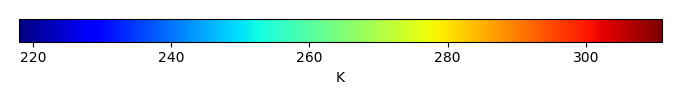MODEL MEANBIAS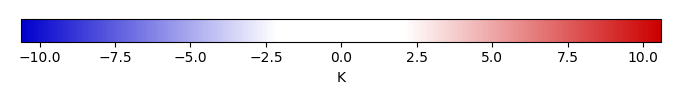BIAS SCORERMSE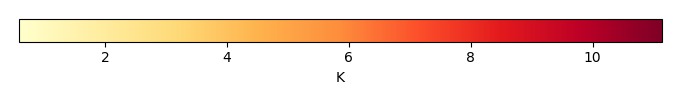RMSE SCOREBENCHMARK INTERANNUAL VARIABILITY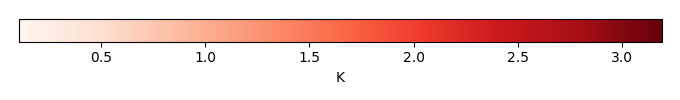MODEL INTERANNUAL VARIABILITYINTERANNUAL VARIABILITY SCOREBENCHMARK MAX MONTHMODEL MAX MONTHDIFFERENCE IN MAX MONTH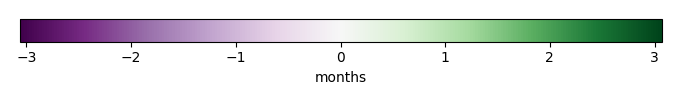SEASONAL CYCLE SCORESPATIAL TAYLOR DIAGRAMMODEL COLORS# Spatially integrated regional mean

MODEL COLORSREGIONAL MEANANNUAL CYCLEMONTHLY ANOMALYANNUAL CYCLE# All Models

BenchmarkCRUNCEPv7GSWP3v1# Data Information

creation_date: Fri Feb 8 14:12:14 PST 2019

history: 2018-01-15: download from https://crudata.uea.ac.uk/cru/data/hrg/cru_ts_4.01/cruts.1709081022.v4.01/ 2019-02-06: converted and created to netCDF4 for ILAMB package

original_resolution: 0.5x0.5

resolution: 0.5x0.5

original_frequency: mon

frequency: mon

references: Harris et al., 2014, Int. J. Climatol., doi:10.1002/joc.3711

institution: Original Data held at British Atmospheric Data Centre, RAL, UK.

source: Run ID = 1709081022. Data generated from:TMX derived from gridded TMP & DTR absolutes, DTR in turn derived from tmn.1708171135.dtb, tmx.1708171215.dtb

title: CRU TS4.01 Maximum Temperature

Conventions: CF-1.7